CONIC SECTIONS - THE PARABOLA

Example 1:

Find the coordinates of the focus and the equation of the directrix of the parabola given by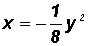.

First, we will convert the equation of the parabola to standard form.

Since there is a y-term raised to the second power we know that this parabola is of the form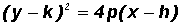.

NOTE: The vertex (h, k) of this parabola is at (0, 0) because there is only a y2-term and no y-term.

Let's multiply both sides of the original equation by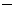8 to change the coefficient of y2 to 1.Now, we can write the equation as follows: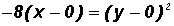The standard form contains a 4p. Currently,8 sits in its position. Therefore, we can say that8 = 4p

and p =2

We can now write the standard form of the original parabolic equation as follows: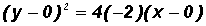where h = 0, k = 0, and p =2

Coordinates of the Focus (h + p, k)

(02, 0) = (2, 0)

Equation of the Directrix x = hp

x = 0(2)

x = 2

Example 2:

Find the coordinates of the focus and the equation of the directrix of the parabola given by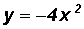.

First, we will convert the equation of the parabola to standard form.

Since there is an x-term raised to the second power we know that this parabola is of the form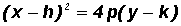.

NOTE: The vertex (h, k) of this parabola is at (0, 0) because there is only an x2-term and no x-term.

Let's divide both sides of the original equation by4 to to change the coefficient of x2 to 1.Now, we can write the equation as follows:The standard form contains a 4p. Currently,sits in its position. Therefore, we can say thatand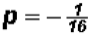We can now write the standard form of the original parabolic equation as follows:where h = 0, k = 0, andCoordinates of the Focus (h, k + p)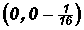=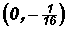Equation of the Directrix y = kp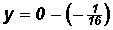andExample 3:

Find the coordinates of the vertex and the focus and the equation of the directrix of the parabola given by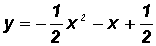.

First, we will convert the equation of the parabola to standard form.

Since there is an x-term raised to the second power we know that this parabola is of the form.

Let's first multiply both sides of the original equation by2 to change the coefficient of x2 to 1.

Then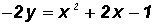.

Next, we'll isolate the terms containing x on one side of the equation as follows.We need (xh)2 on the right! We will use the Square Completion Method to alter the right side appropriately.

We will utilize the term 2x. We divide its coefficient 2 by 2, then we raise this quotient to the second power,

That is,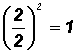.

Now, we add this 1 to BOTH sides of the equation.

12y + 1 = x2 + 2x + 1

andContinuing, we get22y = (x + 1)2

Now we can begin to rewrite this equation into standard form.

We have (x + 1)2, but we can write this as [x(1)]2.This is (xh)2.

We have 22y, but we can write this as2(y1).

The standard form contains a 4p. Currently,2 sits in its position. Therefore, we can say that2 = 4p

then p =We can now write the standard form of the original parabolic equation as follows:

[x(1)]2 = 4()(y1) where h =2, k = 1, and p =Coordinates of the Vertex (h, k)

(1, 1)

Coordinates of the Focus (h, k + p)

(1, 1) = (1,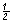)

Equation of the Directrix y = kp

y = 1() = 1 +andExample 4:

Find the coordinates of the vertex and focus and the equation of the directrix of the parabola given by.

First, we will convert the equation of the parabola to standard form.

Since there is a y-term raised to the second power we know that this parabola is of the form.

Let's first multiply both sides of the original equation by 4 to change the coefficient of y2 to 1.

Then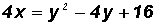.

Next, we'll isolate the terms containing y on one side of the equation as follows.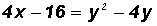We need (yk)2on the right! We will use the Square Completion Method to alter the right side appropriately.

We will utilize the term4y. We divide its coefficient4 by 2, then we raise this quotient to the second power,

That is,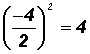.

Now, we add this 4 to BOTH sides of the equation.

4x16 + 4 = y24y + 4

and 4x12 = y24y + 4

Continuing, we get 4x12 = (y2)2 .

Now we can begin to rewrite this equation into standard form.

We have (y2)2 which is (yk)2.

We have 4x12, but we can write this as 4(x3).

The standard form contains a 4p. Currently, 4 sits in its position. Therefore, we can say that

4 = 4p

then p = 1

We can now write the standard form of the original parabolic equation as follows: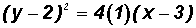where h = 3, k = 2, and p = 1

Coordinates of the Focus (h + p, k)

(3 + 1, 2) = (4, 2)

Equation of the Directrix x = hp

x = 31

and x = 2